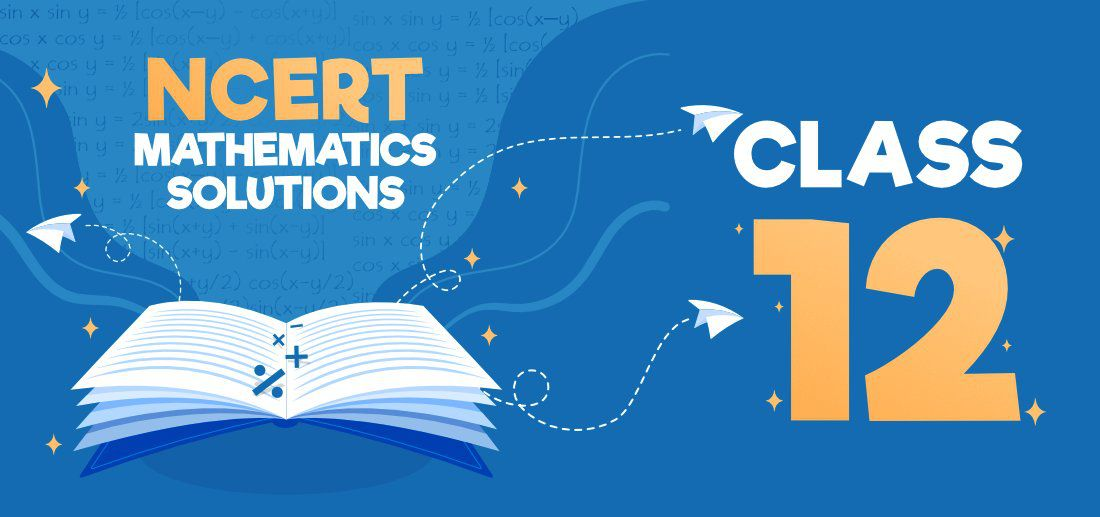GFG App
Open AppBrowser
Continue

# NCERT Solutions for Class 12 Maths

NCERT Solutions for Class 12 Maths provides a solid conceptual base for all the topics included in CBSE Class 12 Maths Syllabus. NCERT Solutions covers all the important theorems and formulae with detailed explanations to promote a better conceptual understanding in students. Our Maths solution can help students like you build a deeper understanding of concepts like Matrices & Determinants, Integrals, Differentials, trigonometric functions, inverse trigonometric, etc.Class 12 Maths NCERT Solutions includes all the answers to problems found in the NCERT Class 12th textbook. These NCERT solutions can be used as a reference by students if they get stuck when answering issues, or as a source to clarify topics. Maths experts meticulously create these NCERT solutions for Class 12 mathematics to guarantee that any student, regardless of intellect level, may easily comprehend the solutions.

## NCERT Solutions for Class 12 Maths Chapters List

The below table contains the NCERT solutions chapter-wise list for Class 12 Maths:-

## Class 12 Maths NCERT Solutions Chapter 1 – Relations and Functions

This section covers NCERT Solutions for Class 12 Maths Chapter 1 – Relation and functions and discusses the introduction of relations and functions, types of relations, types of functions, the composition of functions and invertible functions, binary operations, and miscellaneous examples.

## Class 12 Maths NCERT Solutions Chapter 2 – Inverse Trigonometric Functions

Inverse Trigonometric Functions of NCERT Class 12 Maths Solutions, gives an account of various topics such as the remarks based on basic concepts of inverse trigonometric functions, properties of inverse trigonometric functions, and miscellaneous examples.

## Class 12 Maths NCERT Solutions Chapter 3 – Matrices

In Chapter 3 of the Class 12 Maths NCERT, we shall see the definition of a matrix, types of matrices, equality of matrices, operations on matrices such as the addition of matrices and multiplication of a matrix by a scalar, properties of matrix addition, properties of scalar multiplication, multiplication of matrices, properties of multiplication of matrices, transpose of a matrix, symmetric and skew-symmetric matrices, elementary operation or transformation of a matrix, the inverse of a matrix by elementary operations and miscellaneous examples.

## Class 12 Maths NCERT Solutions Chapter 4 – Determinants

Chapter 4 of Class 12 Maths Solutions discusses the topic of Determinants. Students will get to learn about the definition and meaning of determinants, remarks based on the order of determinants, properties of determinants, finding the area of a triangle using determinants, minors, and cofactors of determinants, adjoint of a matrix, the inverse of a matrix, applications of determinants, and matrices and miscellaneous examples.

## Class 12 Maths NCERT Solutions Chapter 5 – Continuity and Differentiability

Chapter 5 of Class 12 Maths Solutions starts with the definition of Continuity. Students go on to learn about continuity, the algebra of continuous functions, the definition, and meaning of differentiability, derivatives of composite functions, derivative of implicit functions, the derivative of inverse trigonometric functions, exponential and logarithmic functions, logarithmic differentiation, derivatives of functions in parametric forms, second-order derivatives, mean value theorem via various examples.

## Class 12 Maths NCERT Solutions Chapter 6 – Applications of Derivatives

Chapter 6 of the Class 12 Maths Solutions provides a definition of Derivatives, rate of change of quantities, increasing and decreasing functions, tangents and normals, approximations, maxima and minima, first derivative test, maximum and minimum values of a function in a closed interval, and miscellaneous examples.

## FAQs on NCERT Solutions for Class 12 Maths

### Q1: Which book is best for Class 12 Boards’ preparation for Maths?

The NCERT textbook, which is recommended by CBSE, is the best book for not only Class 12 Mathematics but any class. Practice NCERT maths book examples and exercise questions to develop the conceptual foundation needed to tackle HOTs (High Order Thinking Problems).